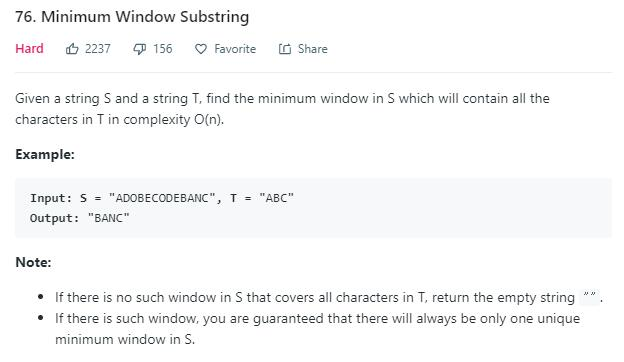# 题目描述（困难难度）# 解法一 滑动窗口

1. right 向右移增大窗口，直到窗口包含了所有要求的字母。进行第二步。
2. 记录此时的长度，left 向右移动，开始减少长度，每减少一次，就更新最小长度。直到当前窗口不包含所有字母，回到第 1 步。
S = "ADOBECODEBANC", T = "ABC"
A D O B E C O D E B A N C //l 和 r 初始化为 0
^
l
r

A D O B E C O D E B A N C //向后移动 r，扩大窗口
^ ^
l r

A D O B E C O D E B A N C //向后移动 r，扩大窗口
^   ^
l   r

A D O B E C O D E B A N C //向后移动 r，扩大窗口
^     ^
l     r

A D O B E C O D E B A N C //向后移动 r，扩大窗口
^       ^
l       r

//此时窗口中包含了所有字母（ABC），停止移动 r，记录此时的 l 和 r，然后开始移动 l
A D O B E C O D E B A N C
^         ^
l         r

//向后移动 l，减小窗口，此时窗口中没有包含所有字母（ABC），重新开始移动 r，扩大窗口
A D O B E C O D E B A N C
^       ^
l       r

//移动 r 直到窗口包含了所有字母（ABC），
//和之前的长度进行比较，如果窗口更小，则更新 l 和 r
//然后开始移动 l，开始缩小窗口
A D O B E C O D E B A N C
^                 ^
l                 r

//此时窗口内依旧包含所有字母
//和之前的长度进行比较，如果窗口更小，则更新 l 和 r
//继续移动 l，继续缩小窗口
A D O B E C O D E B A N C
^               ^
l               r

//此时窗口内依旧包含所有字母
//和之前的长度进行比较，如果窗口更小，则更新 l 和 r
//继续移动 l，继续缩小窗口
A D O B E C O D E B A N C
^             ^
l             r

//继续减小 l，直到窗口中不再包含所有字母，然后开始移动 r，不停的重复上边的过程，直到全部遍历完


public String minWindow(String s, String t) {
Map<Character, Integer> map = new HashMap<>();
//遍历字符串 t，初始化每个字母的次数
for (int i = 0; i < t.length(); i++) {
char char_i = t.charAt(i);
map.put(char_i, map.getOrDefault(char_i, 0) + 1);
}
int left = 0; //左指针
int right = 0; //右指针
int ans_left = 0; //保存最小窗口的左边界
int ans_right = -1; //保存最小窗口的右边界
int ans_len = Integer.MAX_VALUE; //当前最小窗口的长度
//遍历字符串 s
while (right < s.length()) {
char char_right = s.charAt(right);
//判断 map 中是否含有当前字母
if (map.containsKey(char_right)) {
//当前的字母次数减一
map.put(char_right, map.get(char_right) - 1);
//开始移动左指针，减小窗口
while (match(map)) { //如果当前窗口包含所有字母，就进入循环
//当前窗口大小
int temp_len = right - left + 1;
//如果当前窗口更小，则更新相应变量
if (temp_len < ans_len) {
ans_left = left;
ans_right = right;
ans_len = temp_len;
}
//得到左指针的字母
char key = s.charAt(left);
//判断 map 中是否有当前字母
if (map.containsKey(key)) {
//因为要把当前字母移除，所有相应次数要加 1
map.put(key, map.get(key) + 1);
}
left++; //左指针右移
}
}
//右指针右移扩大窗口
right++;
}
return s.substring(ans_left, ans_right+1);
}

//判断所有的 value 是否为 0
private boolean match(Map<Character, Integer> map) {
for (Integer value : map.values()) {
if (value > 0) {
return false;
}
}
return true;
}


public String minWindow(String s, String t) {
int[] map = new int;
// 遍历字符串 t，初始化每个字母的次数
for (int i = 0; i < t.length(); i++) {
char char_i = t.charAt(i);
map[char_i]++;
}
int left = 0; // 左指针
int right = 0; // 右指针
int ans_left = 0; // 保存最小窗口的左边界
int ans_right = -1; // 保存最小窗口的右边界
int ans_len = Integer.MAX_VALUE; // 当前最小窗口的长度
int count = t.length();
// 遍历字符串 s
while (right < s.length()) {
char char_right = s.charAt(right);

// 当前的字母次数减一
map[char_right]--;

//代表当前符合了一个字母
if (map[char_right] >= 0) {
count--;
}
// 开始移动左指针，减小窗口
while (count == 0) { // 如果当前窗口包含所有字母，就进入循环
// 当前窗口大小
int temp_len = right - left + 1;
// 如果当前窗口更小，则更新相应变量
if (temp_len < ans_len) {
ans_left = left;
ans_right = right;
ans_len = temp_len;
}
// 得到左指针的字母
char key = s.charAt(left);
// 因为要把当前字母移除，所有相应次数要加 1
map[key]++;
//此时的 map[key] 大于 0 了，表示缺少当前字母了，count++
if (map[key] > 0) {
count++;
}
left++; // 左指针右移
}
// 右指针右移扩大窗口
right++;
}
return s.substring(ans_left, ans_right + 1);
}